# DAV Class 8 Maths Chapter 15 Worksheet 1 Solutions

The DAV Class 8 Maths Solution and DAV Class 8 Maths Chapter 15 Worksheet 1 Solutions of Statistics and Probability offer comprehensive answers to textbook questions.

## DAV Class 8 Maths Ch 15 WS 1 Solutions

Question 1.
Given below are the ages of 30 students of class-VIII in a school: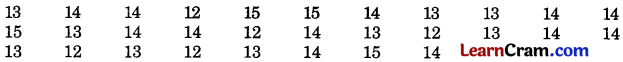Prepare a frequency distribution table.
Solution:Question 2.
The following data gives the number of children in 40 families:Solution:Question 3.
The daily wages (in ₹) of 15 workers in a factory are the following:
300 250 200 250 200 250 200 150 350 200 150 300 150 200 250
Prepare a frequency distribution table. Also answer:
(i) What is the range of wages (in ₹) ?
(ii) How many workers are getting ₹ 300?
(iii) How many workers are getting the maximum wages?
Solution:(i) The range of wages is from ₹ 150 to ₹ 350.
(ii) The number of workers getting ₹ 300 = 2.
(iii) The number of workers getting the maximum wages 1.Question 4.
The daily minimum temperature (in °C) in Delhi for the 30 days of June in a particular ‘ year was as under:Solution:Maximum frequency = 13
Its class interval = 25.5 – 27.0
Class size = 1.5
Class mark = $$\frac{25.5+27}{2}$$
= $$\frac{52.5}{2}$$ = 26.25.Question 5.
(i) What information is depicted by the histogram?
(ii) In which age group is the number of literate females maximum?
(iii) In which age group is the number of literate females minimum?
(iv) How many females below 35 years of age are literate?
Solution: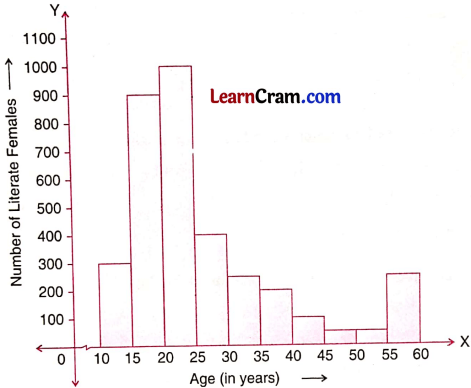(i) The above histogram depicts the information about the age wise number of literacy of females.
(ii) 20 – 25 years
(iii) 45 – 55 years
(iv) In the age group 10 – 15, the literate female are 300.
In the age group 15 – 20, the literate female are 900.
In the age group 20 – 25, the literate female are 1050.
In the age group 25 – 30, the literate female are 400.
In the age group 30 – 35, the literate female are 200.
\frac{25.5+27}{2} Total number of literate female below 35 year are = 300 + 900 + 1050 + 400 + 200 = 2850.Question 6.
Given below is the histogram depicting the marks obtained by 40 students of Class-VIII in a test of English. Look at it carefully and answer the questions that follow:
(i) What is the class size?
(ii) How many students obtained less than 10 marks?
(iii) How many students obtained more than 15 but less than 30 marks?Solution:
(i) Class size is 5.
(ii) The number of students who obtained less than 10 mark is 8 i.e. in 0-5 it is 2 and in 5-10, it is 6.
So the total number = 2 + 6 = 8
(iii) 15 – 20, the number of students = 4
20 – 25, the number of students = 8
25 – 30, the number of students = 10
∴ Total number of students = 4 + 8 + 10 = 22.

Question 7.
The pulse rate (per minute) of 30 persons was recorded as: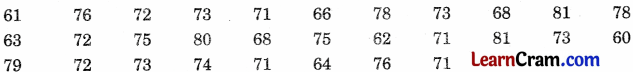Construct a frequency table using class intervals 60-65, 65-70 etc. Answer the following.
(i) What is the upper limit of third class interval?
(ii) What are the class marks of each class interval?
(iii) Which class interval has the highest frequency?
Solution:(i) The upper limit of 70 – 75 is 75.
(ii) Class marks of the intervals 60 – 65, 65 – 70, 70 – 75, 75 – 80, and 80 – 85 are 62.5, 67.5, 72.5, 77.5 and 82.5 respectively.
(iii) 70 – 75 has the highest frequency 14.Question 8.
The electricity bills (in rupees) of 25 houses of a certain locality for a month are given below: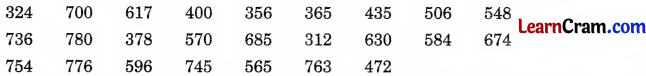Solution: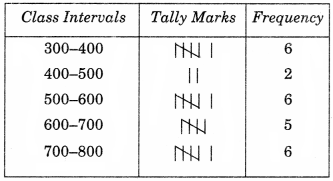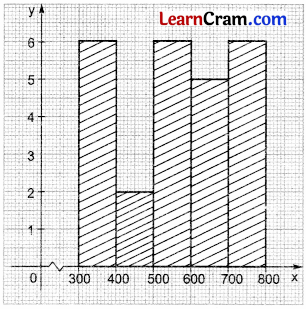Question 9.
There are 40 students in a class. There ages are as follows. Construct a frequency distribution table with one of the class intervals 5-10. Draw histogram also.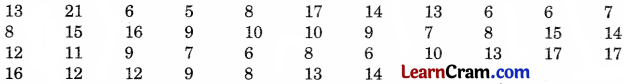Solution: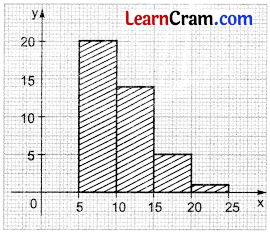Question 10.
Yearly savings (in rupees) of 30 students of class VII are given below. Prepare a frequency distribution table with one of the class interval 30 – 40. Also prepare a histogram.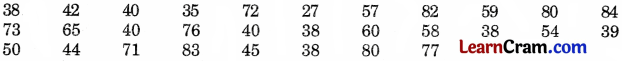Solution: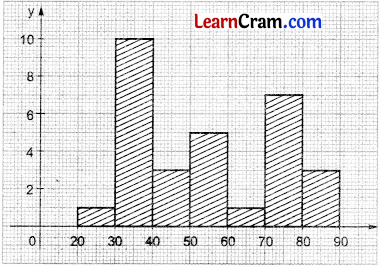### DAV Class 8 Maths Chapter 15 Worksheet 1 Notes

Raw Data:
Collection of all the observations is called raw data.

Range:
The difference between the highest value and the lowest value of the given observations is called range.

Frequency:
The number of times a particular observation occurs in a data is called its frequency.

Grouped frequency distribution:
Raw data are conveniently divided into a number of groups and count the observations falling in each group. This presentation of raw data is called grouped frequency distribution. These groups are called class intervals.

Probability:
Probability is chance of happening and not happening an event.

Probability of an event, P(A) = Number of outcomes / Total number of possible outcomes
P(A) = $$\frac{n(\mathrm{E})}{n(\mathrm{~S})}$$
0 ≤ P(A) ≤ 1
If P(A) = 0, then the event is impossible to occur.
If P(A) = 1, then the event is sure to happen.
Probability of an event is never negative.

Sample Space: Total number of possible outcomes represented by n(S).

Examples:
1. Tossing a coin: n(S) = 2 i.e. H, T
2. Rolling a die: n(S) = 6 i.e. 1, 2, 3, 4, 5, 6Example 1.
Prepare a frequency distribution table with class-size 10 of the given raw data. Also find its range:
24, 36, 54, 8, 6, 12, 18, 6, 9, 25, 32, 39. 48, 45, 42, 31, 20,
16, 37, 27, 19, 11, 7, 3, 14, 18, 50, 34, 23, 13, 50, 56, 4, 52
Solution:Example 2.
Look at the given frequency distribution table and answer the questions given below: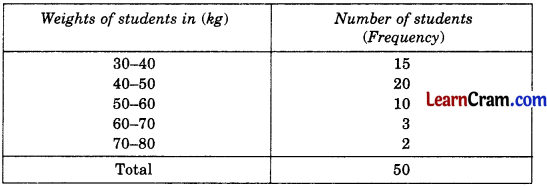(i) What is the class size?
(ii) What is the range?
(iii) Which class has highest frequency?
(iv) Which class has the lowest frequency?
(v) How many students are below 50 kg?
(vi) How many students are above 50 kg?
(vii) How many students are below 50 kg and above 40 kg?
(viii) How many students are below 80 kg and above 80 kg?
Solution:
(i) Class-size is 10.
(ii) Range is from 30 kg to 80 kg.
(iv) 40 – 50 has highest frequency (20).
(iv) 70 – 80 has the lowest frequency (2).
(v) 20 + 15 = 35 students are below 50 kg.
(vi) 10 + 3 + 2 = 15 students are above 50 kg.
(vii) 20
(viii) 50 students are below 80 kg and none is above 80 kg.Example 3.
Given below is the frequency distribution of population in lakhs of different cities. Represent it by Histogram.Solution: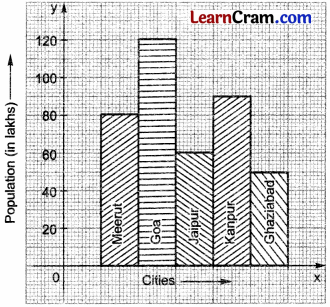Example 4.
A student of class VIII secures the following marks in different subjects given in the table. Draw a pie chart.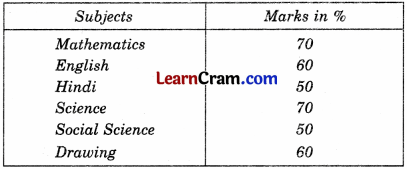Solution:
Let us find the central angle for each subject.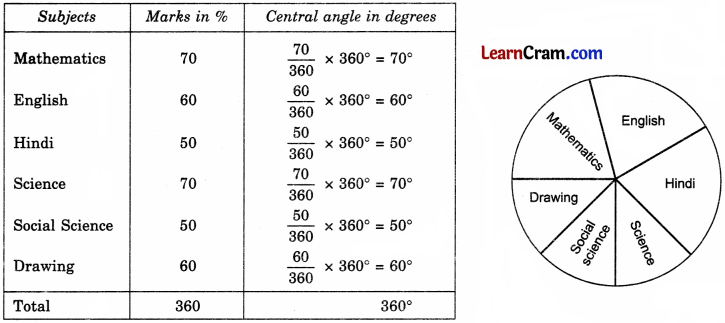Example 5.
A die is thrown once. Find the probability of getting a prime number.
Solution:
Sample Space = 1, 2, 3, 4, 5
∴ n(S) = 6
Getting a prime number i.e. 2, 3, 5 is an event
∴ n(E) = 3
∴ Probability of getting a prime number = $$\frac{n(\mathrm{E})}{n(\mathrm{~S})}=\frac{3}{6}=\frac{1}{2}$$.